Smartick is a fun way to learn math!Nov20

# Singapore Bar Model and Percentages

In today’s post, we’re going to look at percentages through the Singapore bar model.

To define a part of a whole, we can use a fraction, but we can also use a percentage.

The whole divides into 100 equal parts, therefore each part is 1/100 of the whole or 1%.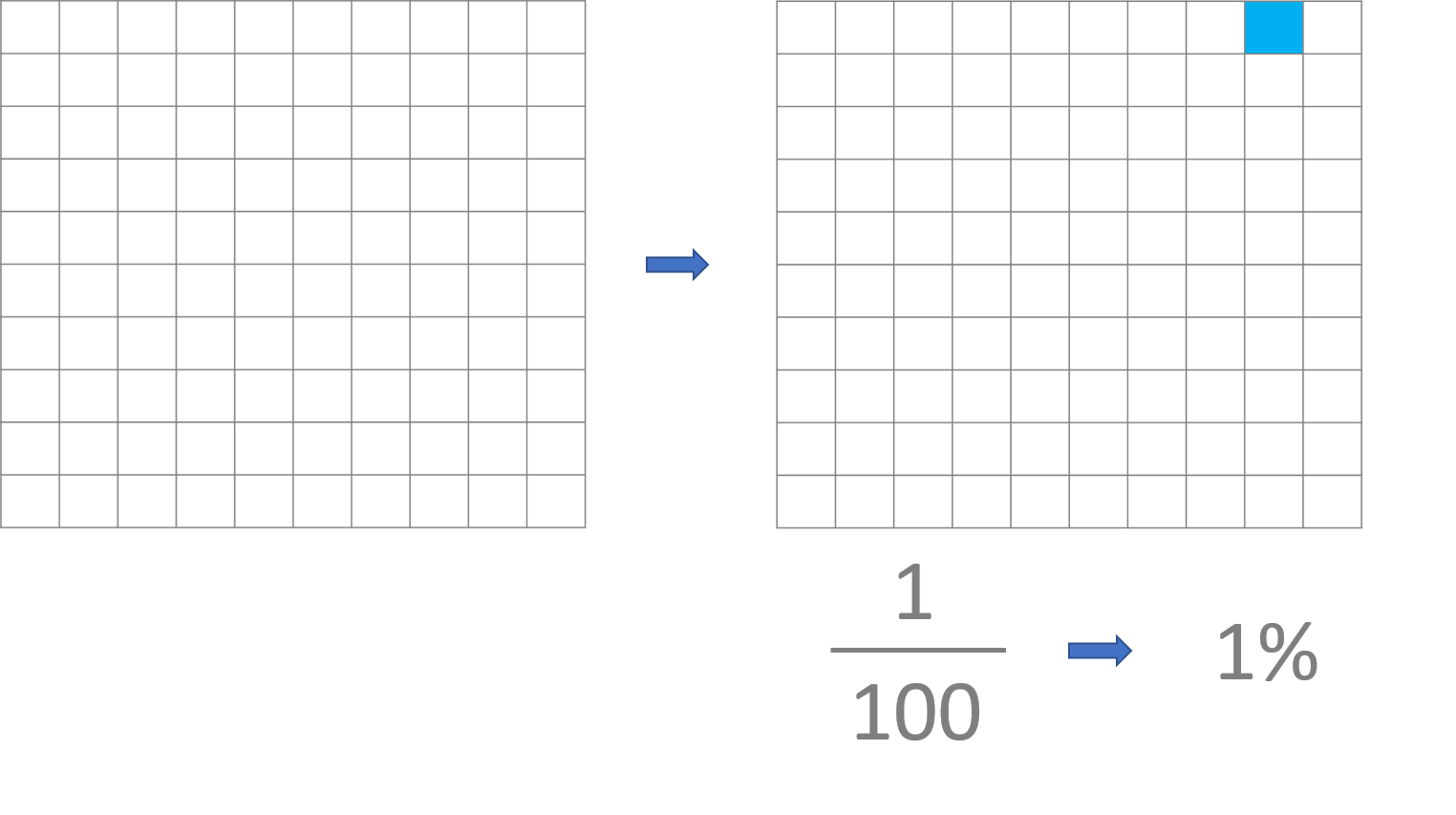#### Let’s look at an example of percentages with the Singapore bar Model:

There are 400 people at a football stadium, and 60% of them are women. How many women are there in total?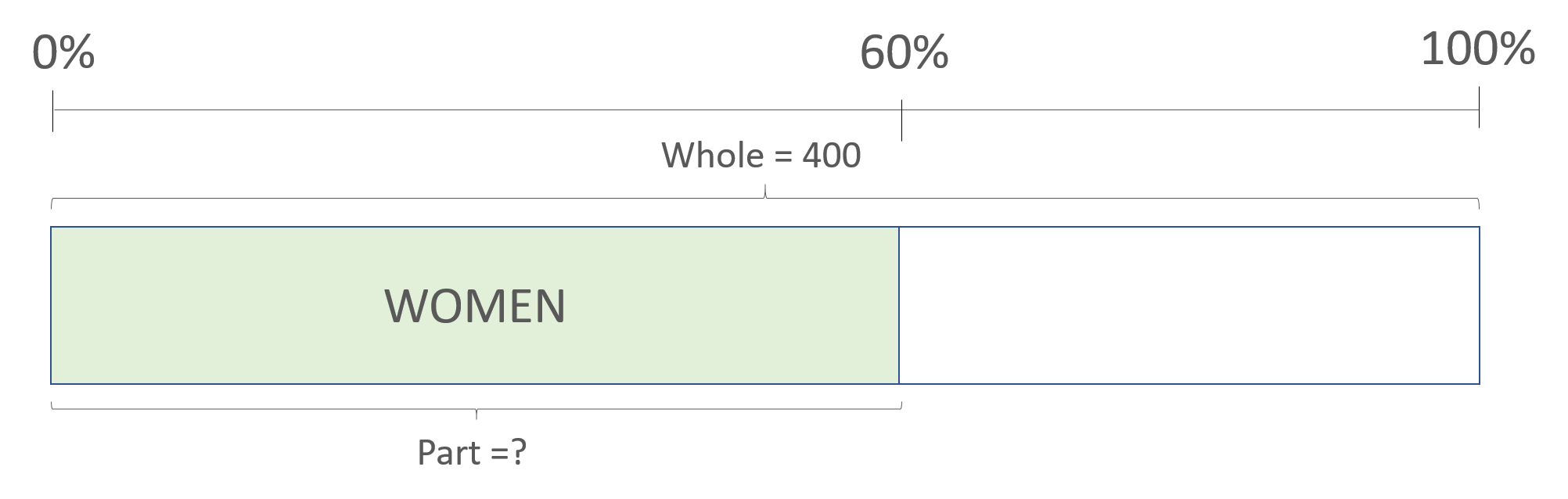In the Singapore bar model, the complete bar represents 100%, therefore the bar is 100 units. The percentage of 60% means that there are 60 units out of 100.

Let’s take a look at how we can work out 60% of 400

We know that in this case, 100 units represents 400 -> 100 units = 400

Therefore, to find out 1 unit -> 1 unit = 400/100 = 4

Now we know that 1 unit is 4 y, and we want to know what 60 units represent -> 60 x 4 = 240

60% of 400 is 240. Therefore, the number of women at the football stadium in total is 240

#### Now, using the same example, we’re going to see how to find the whole when we have just one part:

There are 240 women at a football stadium, which is 60% of the total number of people there. How many people are there in total?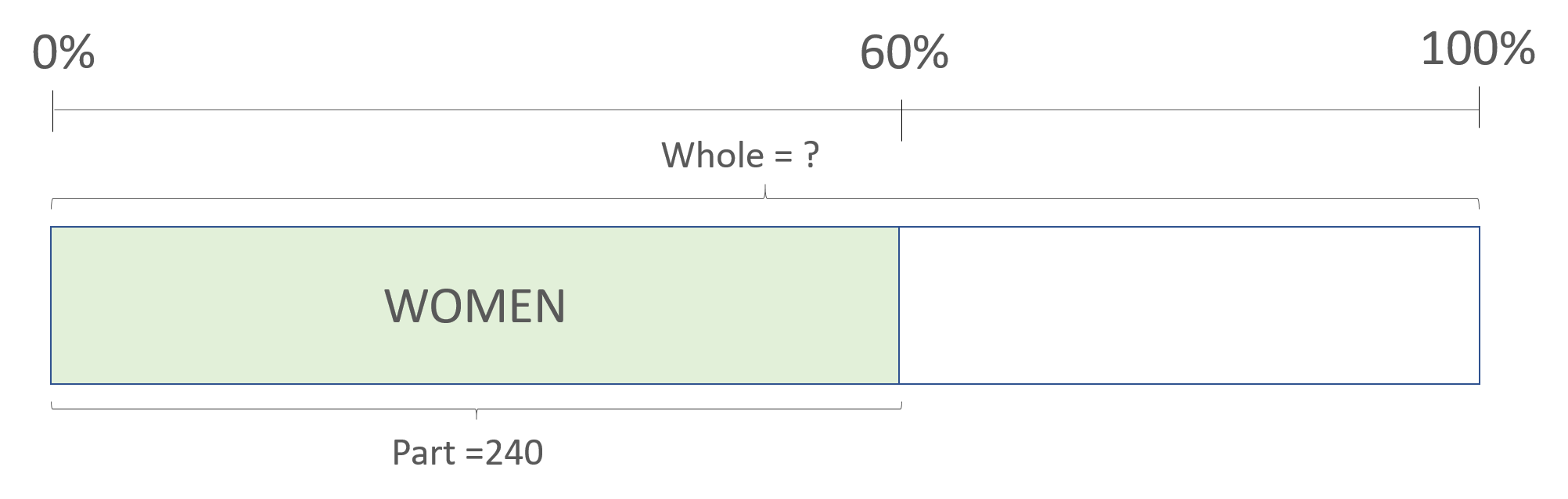We know that 60 units are 240 -> 60 units = 240

Therefore 1 unit is -> 240/60 = 4

We know that 1 unit is 4 and we want to know what 100 units represent->  100 x 4 = 400

The total number of people at the football stadium is 400

#### Let’s look at an example of a comparison problem:

Sam has 70 euros and Tom has 30% more money than Sam. How much money does Tom have?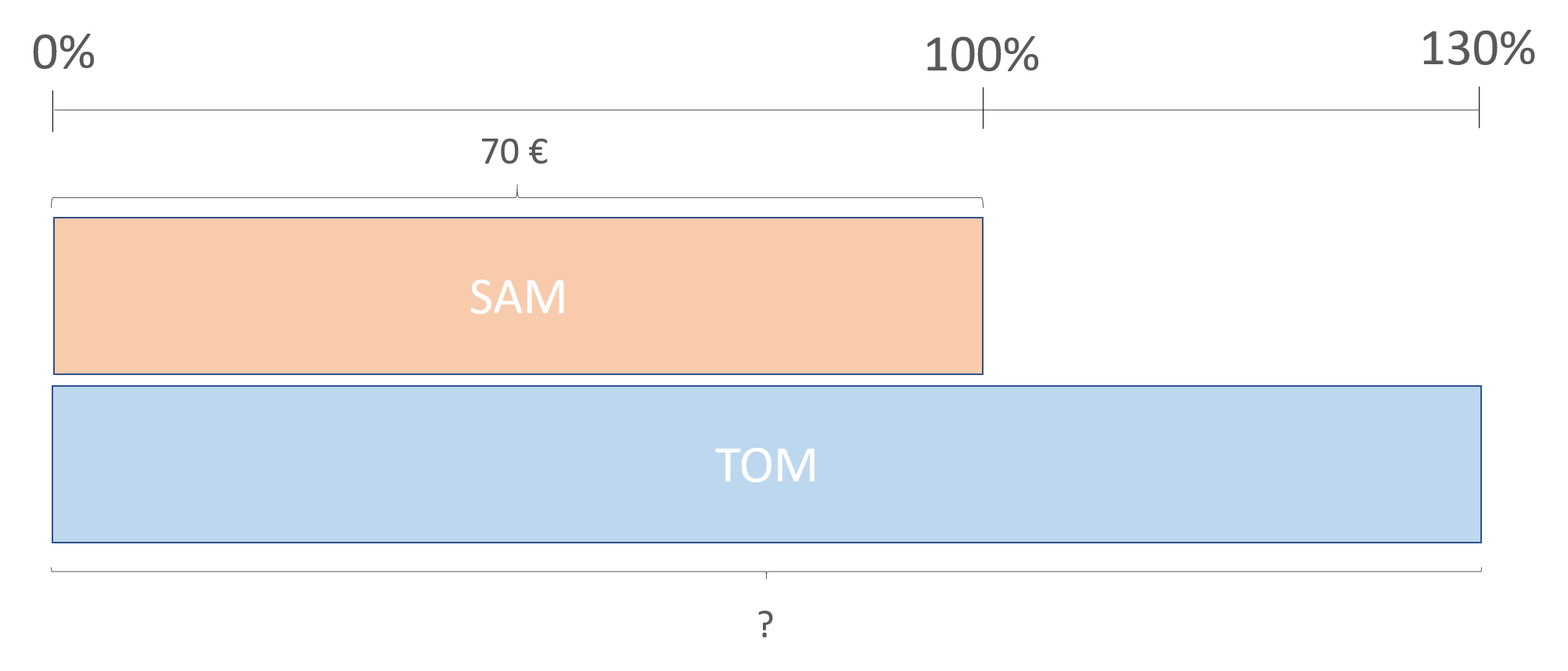In this example, the bar representing Sam’s money acts as a base, 100%, because Tom’s money is based on the relation to the money that Sam has. In this case, Tom has 30% more money than Sam. Therefore, the amount of money Tom has is 130% of the money Sam has. The percentage 130% is 130 units out of 100, and 100 units are equivalent to the €70 that Sam has.

100 units = €70

1 unit = 70/100 = €0.70

130 units = 130 x 0.70 = €91

Therefore, Tom has €91

#### Let’s take a look at another example of comparison:

Julie has 50 books in her library, and Amy has 20% fewer books than Julie. How many books does Amy have in her library?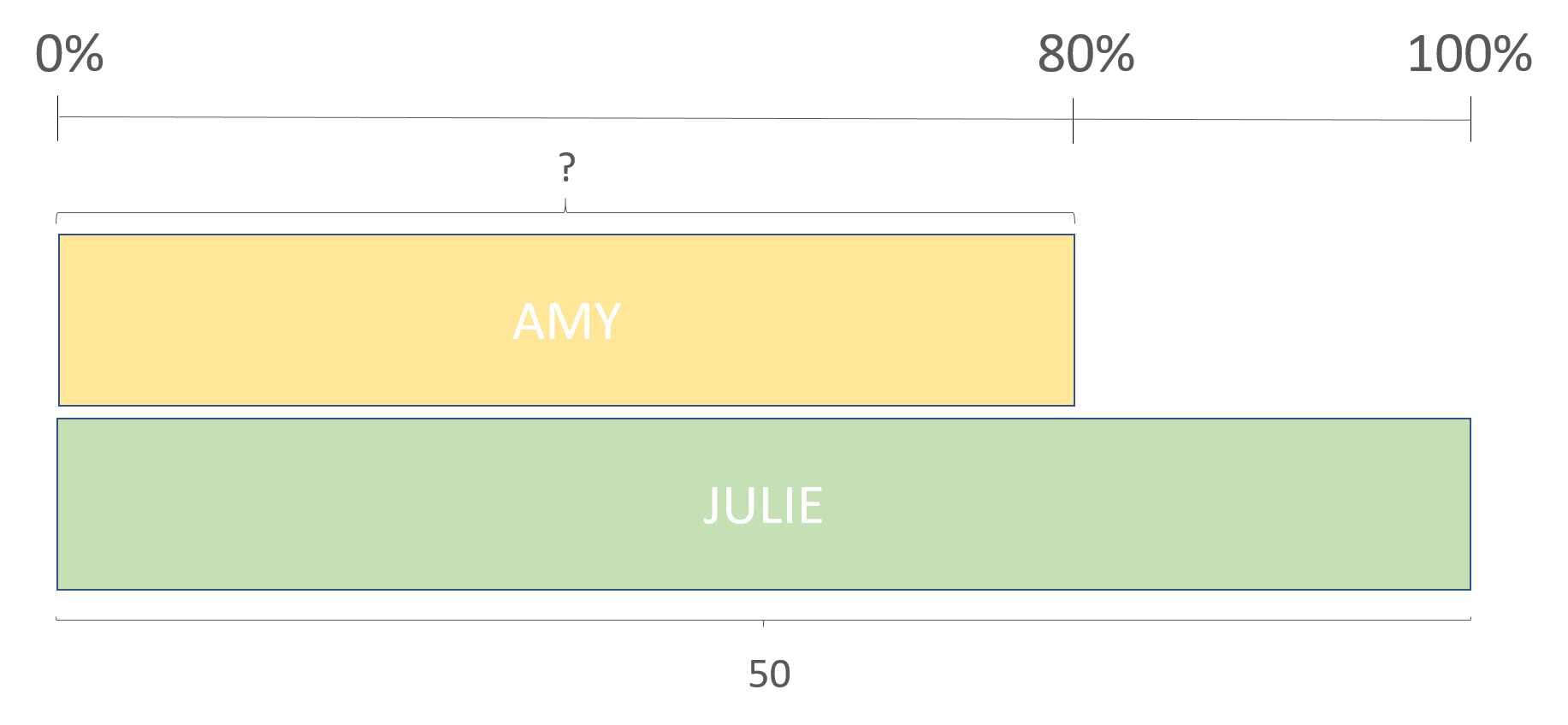We know how many books Julie has, so we can use that amount as a base. Therefore, 50 books are 100%. Amy has 20% fewer books than Julie, therefore, Amy has 80% of the books that Julie has.

100 units = 50 books

1 unit = 50/100 = 0.5

80 units = 80 x 0.5 = 40

Amy has 40 books in her library

I hope you’ve enjoyed learning about the concept of percentages and how to solve them. If you want to keep practicing, log in to Smartick.